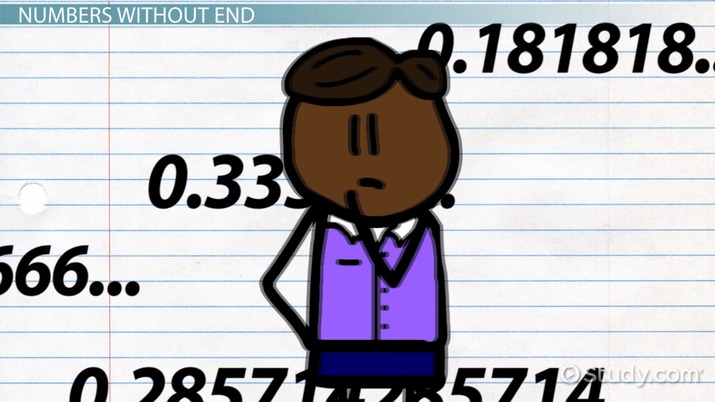# Decimal Expansion of Rational Numbers

Lesson Transcript
Instructor: Thomas Higginbotham

Tom has taught math / science at secondary & post-secondary, and a K-12 school administrator. He has a B.S. in Biology and a PhD in Curriculum & Instruction.

Decimal expansion refers to the repetition or termination of numerals in a decimal number, typically found in rational numbers, those expressible in a fraction or ratio. Learn about finite and repeating decimals and how to convert repeating decimals to rational numbers. Updated: 11/27/2021

## Numbers Without End

Sometimes in math it can feel like the numbers we see go on and on without any real pattern. And sometimes, that's indeed the case. However, more often, those numbers do end up either terminating or forever repeating.

When we express a number as a decimal, it is called decimal expansion. Decimal expansion is the form of a number that has a decimal point, either actual or implied. Examples of numbers with actual decimal points are 10.2 and 0.0084. An example of a number with implied decimal points is the whole number 17, which could actually be written as 17.000000000000. We often leave off the repeating zeros for ease of reading and figuring.

Decimals either terminate or repeat, which is a characteristic of rational numbers. When we use the term rational in math, we're not talking about a number that makes logical sense. Rational numbers can either be written as a fraction of two whole numbers or a ratio.An error occurred trying to load this video.

Try refreshing the page, or contact customer support.

Coming up next: Graphing Rational Numbers on a Number Line

### You're on a roll. Keep up the good work!

Replay
Your next lesson will play in 10 seconds
• 0:00 Numbers Without End
• 1:08 Finite and Repeating Decimals
• 2:37 Converting Repeating…
• 4:10 Lesson Summary
Save Save

Want to watch this again later?

Timeline
Autoplay
Autoplay
Speed Speed

## Finite and Repeating Decimals

Rational decimals that end with repeating zeroes are known as finite decimals, like 6/3 = 2 or 10/2.5 = 4. They're the opposite of infinite or forever. Rational decimals that are finite are those that originate from a fraction with a denominator that is a product of 2, 5, or both. For example, 3/8, 13/25, and 7/50 are all finite decimals because:

8 = 2^3

25 = 5^2

50= 5^2 * 2

Rational decimals that forever repeat are known as repeating decimals. Repeating decimals originate from fractions whose denominators are not entirely products of 2 and 5 only. For example, 3/14 and 7/22 are repeating decimals because 14 = 2 * 7 and 22 = 2 * 11. The number of digits that repeat is referred to as the period of the repeating decimal.

For example:

• Thirds have a period of 1 (1/3 = 0.3333333...)
• Elevenths have a period of 2 (2/11 = 0.1818181818….)
• Sevenths have a period of 6 (2/7 = 0.285714285714285714…..)

## Converting Repeating Decimals to Rational Numbers

If we want to show that a repeating decimal is rational, we can use the following simple steps:

To unlock this lesson you must be a Study.com Member.

### Register to view this lesson

Are you a student or a teacher?